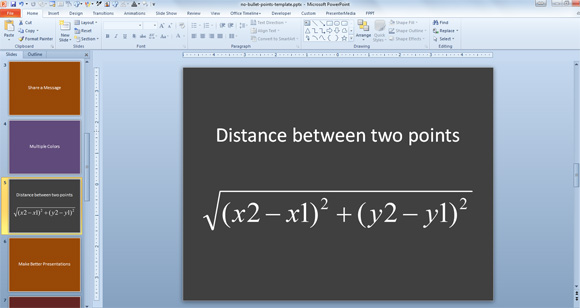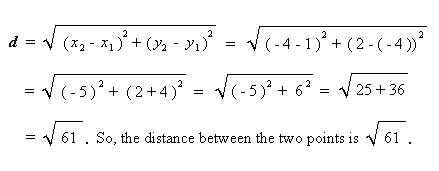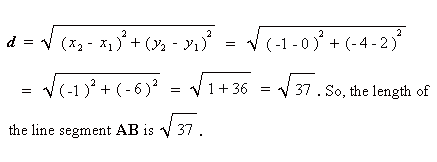# What is the Distance Formula?

The distance between to given points is calculated by using a distance formula.

The distance formula can be helpful if you need to find the distance between to points on the coordinate.### Examples of Euclidean Distance Formula Application

If you need to find the distance between the points on the coordinate system given by ( 1, -4 ) and ( -4, 2 ), then you can apply the distance formula as follows.Another good example is to measure the length of a line using the distance formula. Here we will apply the formula to get the length of a line segment AB where the point A is at ( 0, 2 ) and point B is at ( -1, – 4 ).